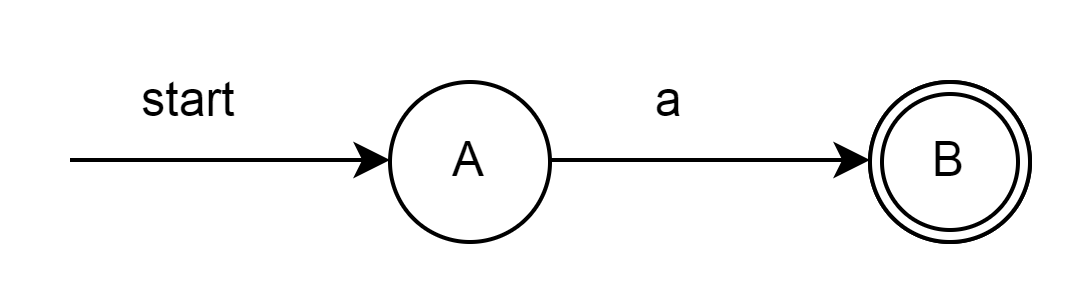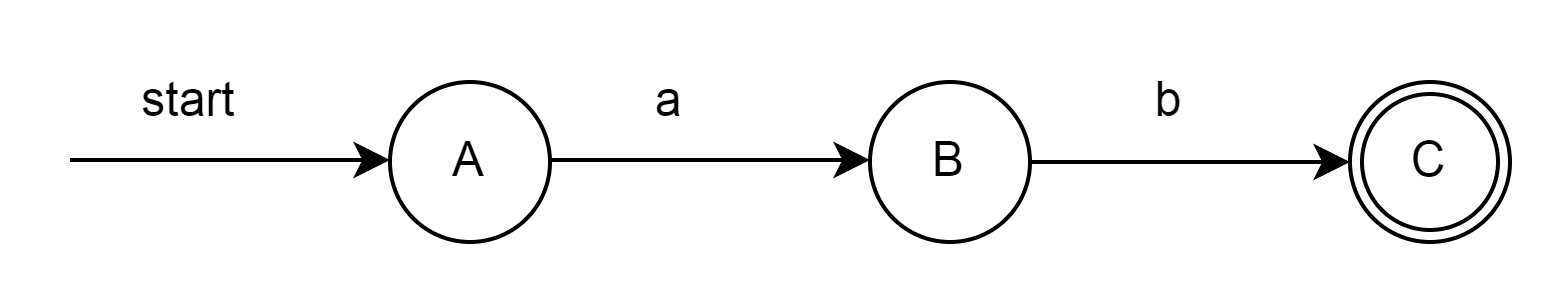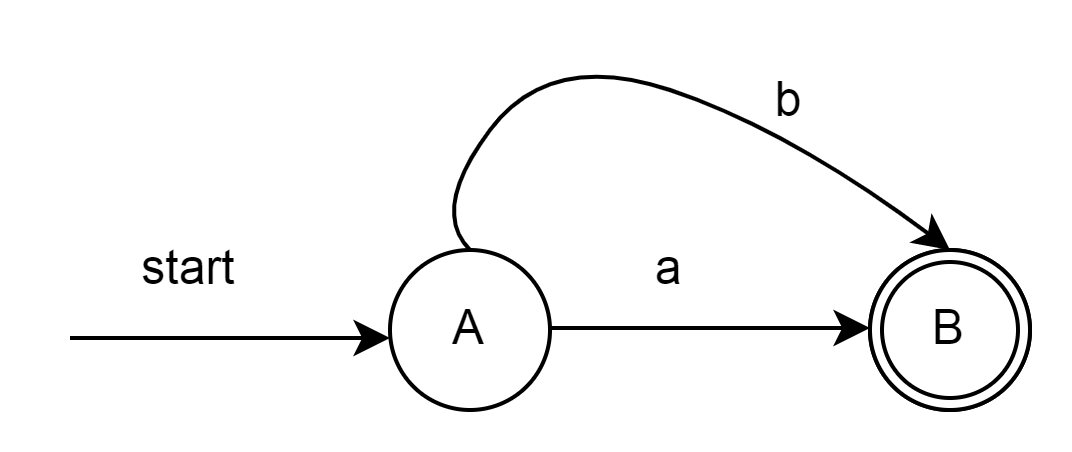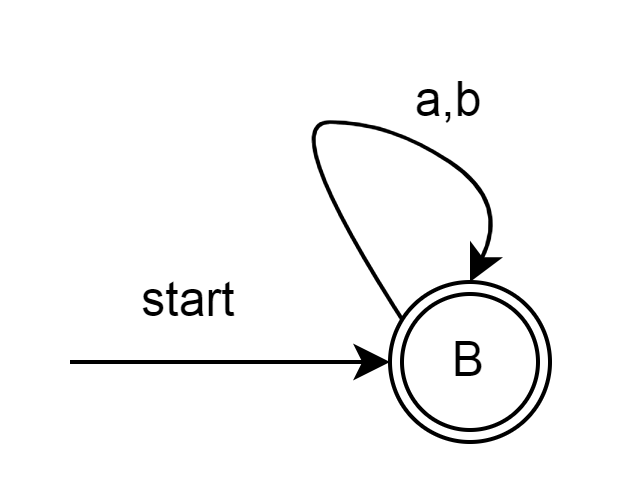### Representation of Finite Automata / Finite state Automata

Transition Graph

• Transition diagram is a graph with nodes representing states and edges representing transition of symbols

• Circles of transition graph represent node

• Directed edges indicate the transition from x to y happens on symbol a, if 𝜹(y , a) = x

• Start state is indicated by an arrow without source

• Final / Accept state is represented by double circle

Transition Table

• Transition table is used to represent the transition function

• The table holds the state and symbols in it

• Table column contains symbols

• Table rows represent the states

• Entries in the table represent the transition to next state

• Start state is indicated by an arrow without source

• Final / Accept state is represented by a star

To start with, let us draw the transition graph for certain simple regular expressions for the alphabet, Σ= {a,b}

1. RE= a1. RE = ab1. RE = a | b1. RE = (a | b) *